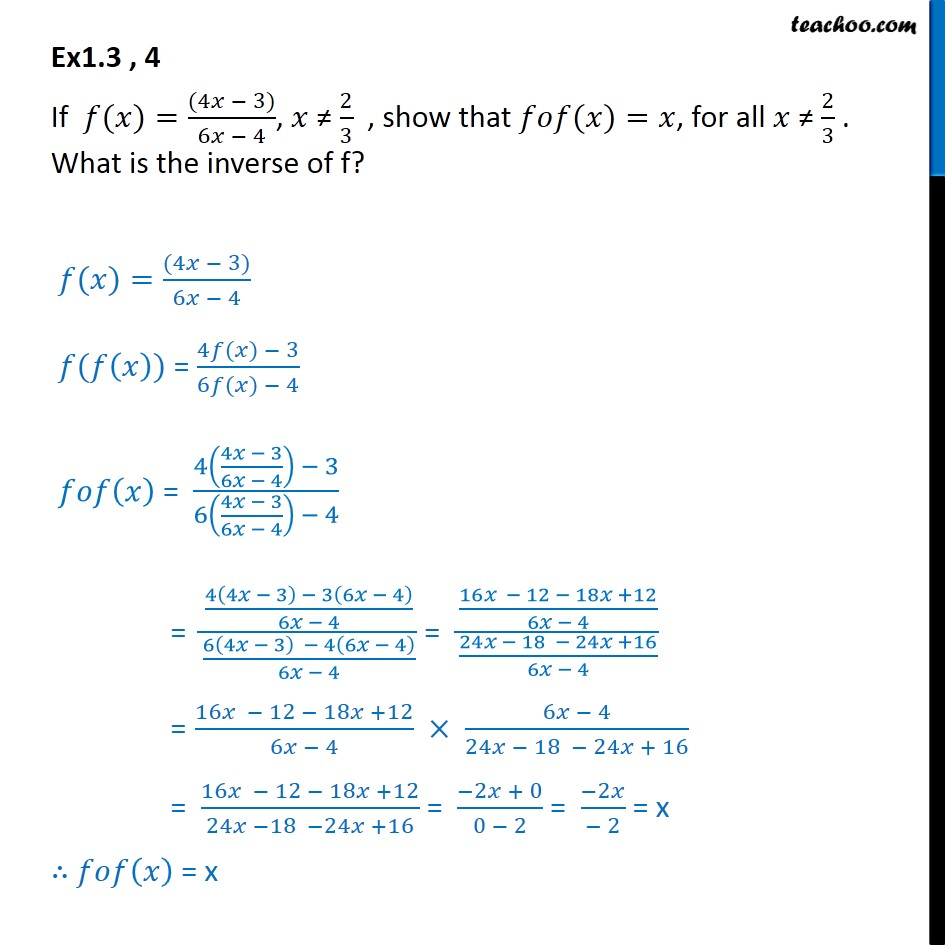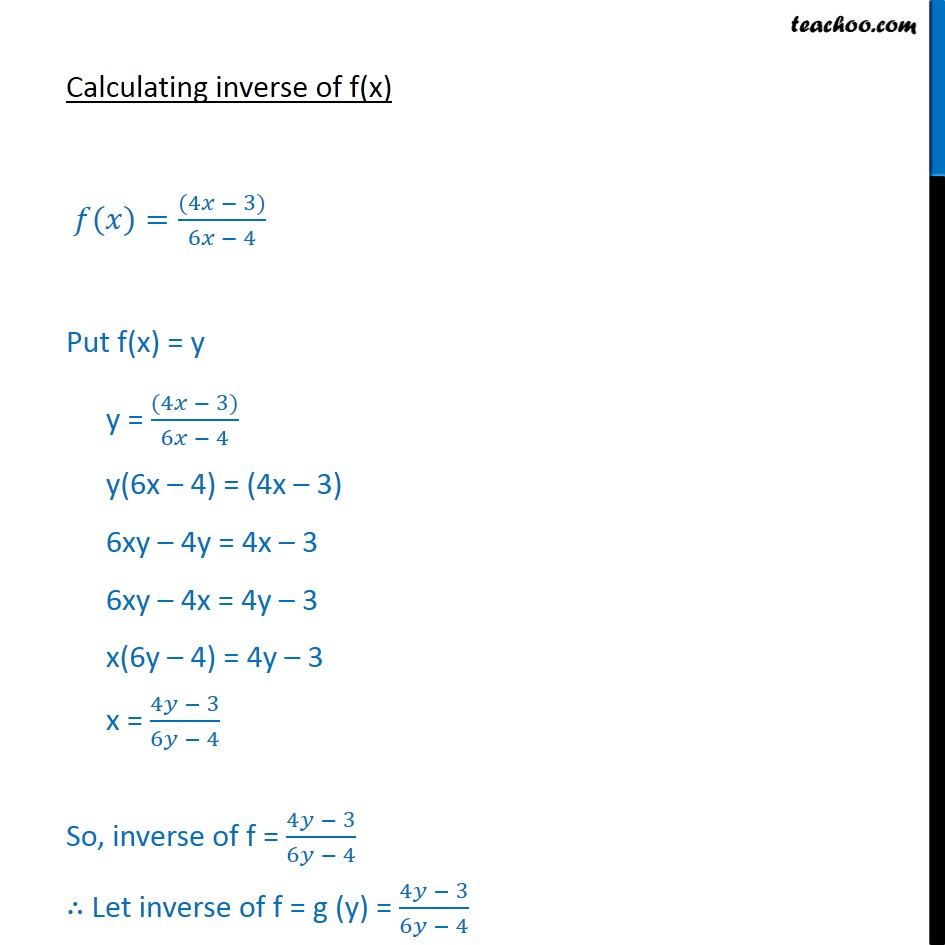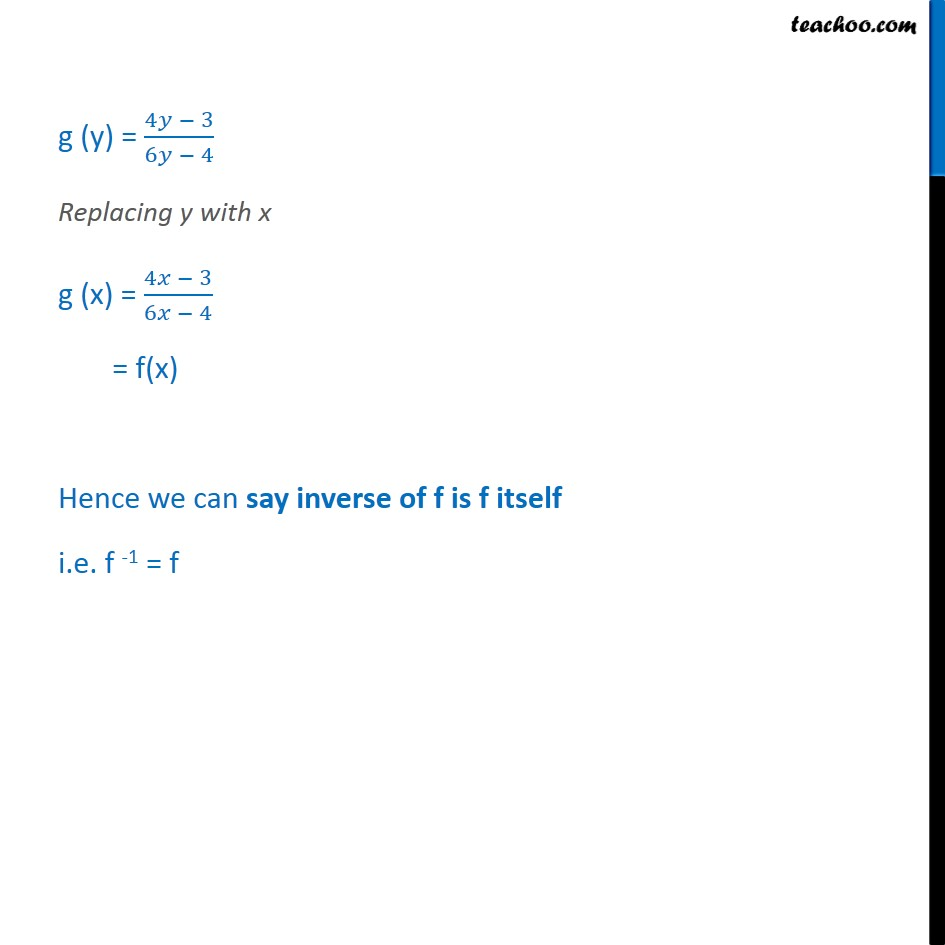Inverse of a function

Chapter 1 Class 12 Relation and Functions
Serial order wiseLearn in your speed, with individual attention - Teachoo Maths 1-on-1 Class

### Transcript

Ex1.3 , 4 If 𝑓(𝑥)=﷐(4𝑥 − 3)﷮6𝑥 − 4﷯, 𝑥 ≠ ﷐2﷮3﷯ , show that 𝑓𝑜𝑓(𝑥)=𝑥, for all 𝑥 ≠ ﷐2﷮3﷯ . What is the inverse of f? 𝑓(𝑥)=﷐(4𝑥 − 3)﷮6𝑥 − 4﷯ 𝑓(𝑓﷐𝑥﷯) = ﷐4𝑓(𝑥) − 3﷮6𝑓(𝑥) − 4﷯ 𝑓𝑜𝑓﷐𝑥﷯ = ﷐4﷐﷐4𝑥 − 3﷮6𝑥 − 4﷯﷯ − 3﷮6﷐﷐4𝑥 − 3﷮6𝑥 − 4﷯﷯ − 4﷯ = ﷐﷐4﷐4𝑥 − 3﷯ − 3﷐6𝑥 − 4﷯﷮6𝑥 − 4﷯﷮﷐6﷐4𝑥 − 3﷯ − 4﷐6𝑥 − 4﷯﷮6𝑥 − 4﷯﷯ = ﷐﷐16𝑥 − 12 − 18𝑥 +12﷮6𝑥 − 4﷯﷮﷐24𝑥 − 18 − 24𝑥 +16﷮6𝑥 − 4﷯﷯ = ﷐16𝑥 − 12 − 18𝑥 +12﷮6𝑥 − 4﷯ × ﷐6𝑥 − 4﷮24𝑥 − 18 − 24𝑥 + 16﷯ = ﷐16𝑥 − 12 − 18𝑥 +12﷮24𝑥 −18 −24𝑥 +16﷯ = ﷐−2𝑥 + 0﷮0 − 2﷯ = ﷐−2𝑥﷮− 2﷯ = x ∴ 𝑓𝑜𝑓﷐𝑥﷯ = x Calculating inverse of f(x) 𝑓(𝑥)=﷐(4𝑥 − 3)﷮6𝑥 − 4﷯ Put f(x) = y y = ﷐(4𝑥 − 3)﷮6𝑥 − 4﷯ y(6x – 4) = (4x – 3) 6xy – 4y = 4x – 3 6xy – 4x = 4y – 3 x(6y – 4) = 4y – 3 x = ﷐4𝑦 − 3﷮6𝑦 − 4﷯ So, inverse of f = ﷐4𝑦 − 3﷮6𝑦 − 4﷯ ∴ Let inverse of f = g (y) = ﷐4𝑦 − 3﷮6𝑦 − 4﷯ g (y) = ﷐4𝑦 − 3﷮6𝑦 − 4﷯ Replacing y with x g (x) = ﷐4𝑥 − 3﷮6𝑥 − 4﷯ = f(x) Hence we can say inverse of f is f itself i.e. f -1 = f